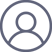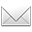李毅伟2019-4-18 16:13

[按：下文是群邮件的内容，标题是新拟的 (原标题“完形”)。]············* * *

In fact, the same ideas work in greater generality.
---- Th1.1的思想适用于更一般的情形.
.
Definition 1.2. A perfectoid field is a complete topological field K whose topology is induced by a nondiscrete valuation of rank 1, such that the Frobenius Φ is surjective on K/p.
---- “完域” 是指 完全拓扑域 K， 其拓扑诱导于 秩1 非离散估值，使得 Frobenius Φ 在 K/p 上是满的.
1. 主集合 K 是完全拓扑域.
2. 用 秩1非离散估值 ndv(1) 给出其拓扑.
3. Frobenius Φ 在 K/p 上是满的.

---- 小波分析里有个“多分辨分析”，也出现了集合与映射方面的约束.
.
Here K ⊂ K denotes the set of powerbounded elements.
---- K 的整元子环是由 有界幂元 构成的集合.
.
Generalizing the example above, a construction of Fontaine associates to any perfectoid field K another perfectoid field K of characteristic p...
---- 推广上述例子，运用 Fontaine 构造，给任何完域 K 关联一个特征p 完域 K ...
.
...whose underlying multiplicative monoid can be described as K = lim<K (x x^p).
---- 其底层 可乘幺半群可描述为 K = lim<K (x x^p).
.

*

1. 对任何 [K]， 预构造 [K]c.
---- [·] 暂标识有限扩张; 下标 c 提示规范.
---- 原作记作 L 及 L#.
.
2. [K] 是 (ak)d 的分裂域，也是 (ak^1/δn)d 的分裂域（n≥0）.
---- (ak)d 标识d阶多项式(首项系数为1).
---- ak 标识系数，k=d-1，...，0.
{补充：这句话体现出的性质非常巧妙(也至关重要).
---- 它也是某种不变性.}

.
3. 只须取 [K]c 为 ((ak^1/δn)#)d 的分裂域（n 足够大）.
.

---- 它起到了 “传递” 的作用(好比几何原本中用圆来传递等长那样).
---- 以上没有变化的还有 形式 [·]，即有限扩张.

---- 则有 (x)d --> (x#)d (好比圆的半径从一个值变化为另一个值).
---- 然后看出，[·] 可类比为 “外接正方形”(其中的 · 可类比为面积之类):
---- ......[K] --> [K]c (这里的下标 c 并没有实质意义).

---- K°/p  K°/p.(para.3a)
---- K = lim<K, x x^p.(para.3b)